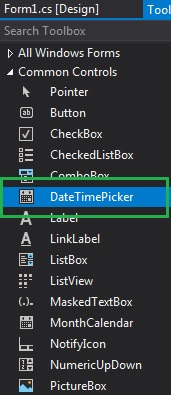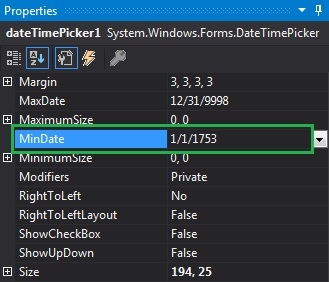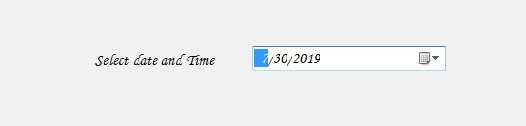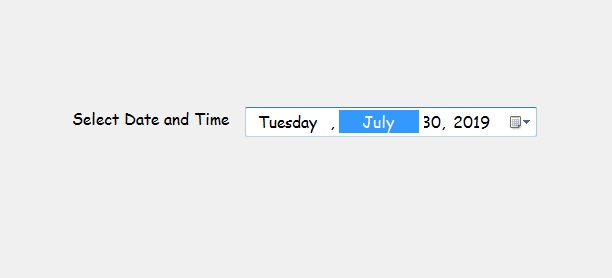# How to set Minimum Date in the DateTimePicker in C#?

• Last Updated : 02 Aug, 2019

In Windows Forms, the DateTimePicker control is used to select and display date/time with a specific format in your form. In DateTimePicker control, you can set the minimum date and time that can be selected in the DateTimePicker using the MinDate Property. The default value of this property is 1/1/1753 00:00:00. You can set this property in two different ways:

1. Design-Time: It is the easiest way to set the minimum date and time in the DateTimePicker as shown in the following steps:

• Step 1: Create a windows form as shown in the below image:
Visual Studio -> File -> New -> Project -> WindowsFormApp• Step 2: Next, drag and drop the DateTimePicker control from the toolbox to the form as shown in the below image:• Step 3: After drag and drop you will go to the properties of the DateTimePicker and set the minimum date and time in the DateTimePicker as shown in the below image:Output:2. Run-Time: It is a little bit trickier than the above method. In this method, you can set the minimum date and time in the DateTimePicker control programmatically with the help of given syntax:

`public DateTime MinDate { get; set; }`

It will throw an ArgumentException if the value of this property is not less than the MaxDate value and also throw a SystemException if the value of this property is less than the MinDateTime value. The following steps show how to set the minimum date and time in the DateTimePicker dynamically:

• Step 1: Create a DateTimePicker using the DateTimePicker() constructor is provided by the DateTimePicker class.
```// Creating a DateTimePicker
DateTimePicker dt = new DateTimePicker();
```
• Step 2: After creating DateTimePicker, set the MinDate property of the DateTimePicker provided by the DateTimePicker class.
```// Setting the minimum date and time
dt.MinDate = new DateTime(1753, 1, 1);
```
• Step 3: And last add this DateTimePicker control to the form using the following statement:
```// Adding this control to the form
```

Example:

 `using` `System;``using` `System.Collections.Generic;``using` `System.ComponentModel;``using` `System.Data;``using` `System.Drawing;``using` `System.Linq;``using` `System.Text;``using` `System.Threading.Tasks;``using` `System.Windows.Forms;`` ` `namespace` `WindowsFormsApp48 {`` ` `public` `partial` `class` `Form1 : Form {`` ` `    ``public` `Form1()``    ``{``        ``InitializeComponent();``    ``}`` ` `    ``private` `void` `Form1_Load(``object` `sender, EventArgs e)``    ``{``        ``// Creating and setting the ``        ``// properties of the Label``        ``Label lab = ``new` `Label();``        ``lab.Location = ``new` `Point(183, 162);``        ``lab.Size = ``new` `Size(172, 20);``        ``lab.Text = ``"Select Date and Time"``;``        ``lab.Font = ``new` `Font(``"Comic Sans MS"``, 12);`` ` `        ``// Adding this control ``        ``// to the form``        ``this``.Controls.Add(lab);`` ` `        ``// Creating and setting the ``        ``// properties of the DateTimePicker``        ``DateTimePicker dt = ``new` `DateTimePicker();``        ``dt.Location = ``new` `Point(360, 162);``        ``dt.Size = ``new` `Size(292, 26);``        ``dt.MaxDate = ``new` `DateTime(2500, 12, 20);``        ``dt.MinDate = ``new` `DateTime(1753, 1, 1);``        ``dt.Format = DateTimePickerFormat.Long;``        ``dt.Name = ``"MyPicker"``;``        ``dt.Font = ``new` `Font(``"Comic Sans MS"``, 12);``        ``dt.Visible = ``true``;``        ``dt.Value = DateTime.Today;`` ` `        ``// Adding this control``        ``// to the form``        ``this``.Controls.Add(dt);``    ``}``}``}`

Output:My Personal Notes arrow_drop_up